# Erdos, Paul

## Multicolour Erdős--Hajnal Conjecture ★★★

Author(s): Erdos; Hajnal

Conjecture   For every fixedand fixed colouringof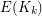withcolours, there exists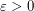such that every colouring of the edges ofcontains eithervertices whose edges are coloured according toorvertices whose edges are coloured with at mostcolours.

Keywords: ramsey theory

## Turán Problem for $10$-Cycles in the Hypercube ★★

Author(s): Erdos

Problem   Bound the extremal number ofin the hypercube.

Keywords: cycles; extremal combinatorics; hypercube

## Erdős–Faber–Lovász conjecture ★★★

Author(s): Erdos; Faber; Lovasz

Conjecture   Ifis a simple graph which is the union ofpairwise edge-disjoint complete graphs, each of which hasvertices, then the chromatic number ofis.

Keywords: chromatic number

## Odd-cycle transversal in triangle-free graphs ★★

Author(s): Erdos; Faudree; Pach; Spencer

Conjecture   Ifis a simple triangle-free graph, then there is a set of at mostedges whose deletion destroys every odd cycle.

Keywords:

## Turán number of a finite family. ★★

Author(s): Erdos; Simonovits

Given a finite familyof graphs and an integer, the Turán number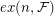ofis the largest integersuch that there exists a graph onvertices withedges which contains no member ofas a subgraph.

Conjecture   For every finite familyof graphs there exists ansuch that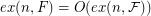.

Keywords:

## Strong edge colouring conjecture ★★

Author(s): Erdos; Nesetril

A strong edge-colouring of a graphis a edge-colouring in which every colour class is an induced matching; that is, any two vertices belonging to distinct edges with the same colour are not adjacent. The strong chromatic index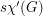is the minimum number of colours in a strong edge-colouring of.

ConjectureKeywords:

## Erdős–Straus conjecture ★★

Author(s): Erdos; Straus

Conjecture

For all, there exist positive integers,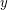,such that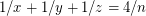.

Keywords: Egyptian fraction

## Double-critical graph conjecture ★★

Author(s): Erdos; Lovasz

A connected simple graphis called double-critical, if removing any pair of adjacent vertexes lowers the chromatic number by two.

Conjectureis the only-chromatic double-critical graph

Keywords: coloring; complete graph

## Erdös-Szekeres conjecture ★★★

Author(s): Erdos; Szekeres

Conjecture   Every set ofpoints in the plane in general position contains a subset ofpoints which form a convex-gon.

## Middle levels problem ★★

Author(s): Erdos

Conjecture   Letbe the bipartite graph whose vertices are the-subsets and the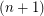-subsets of a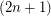-element set, and with inclusion as the adjacency relationship. Thenis Hamiltonian.

Keywords: